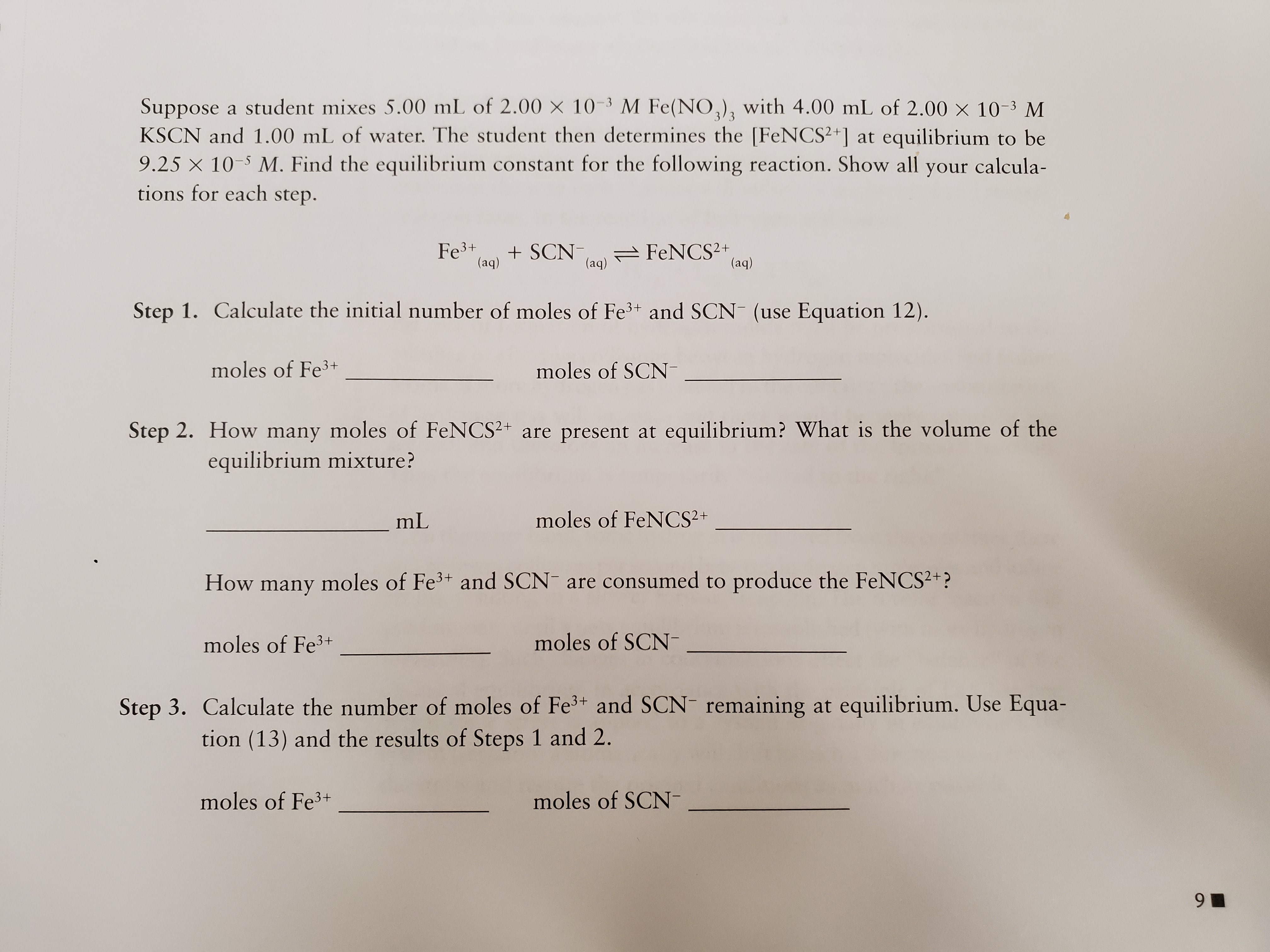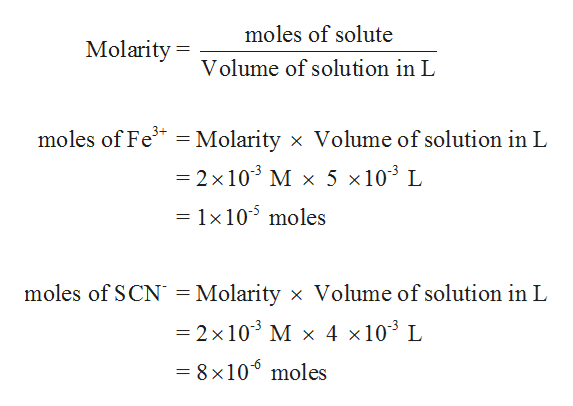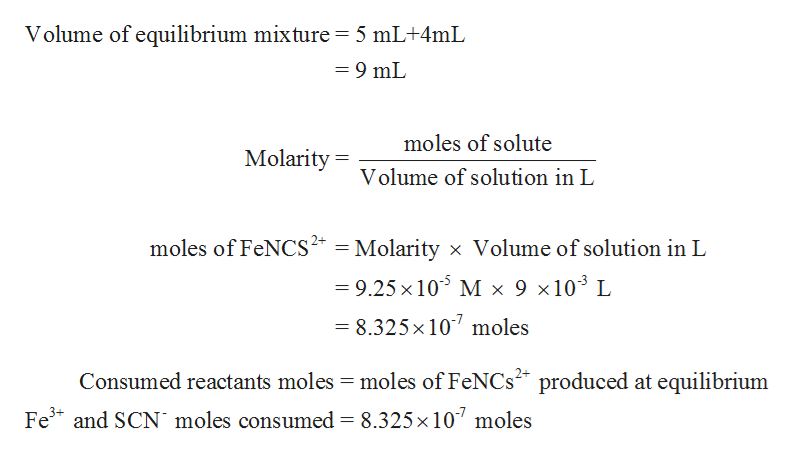Suppose a student mixes 5.00 mL of 2.00 x 10-3 M Fe(NO,)3 with 4.00 mL of 2.00 x 10-3 MKSCN and 1.00 mL of water. The student then determines the [FeNCS2+] at equilibrium to be9.25 X 10 M. Find the equilibrium constant for the following reaction. Show all your calcula-tions for each step.3/3Fe3+(aq)SCN (aq FeNCS2+(aq)Step 1. Calculate the initial number of moles of Fe3+ and SCN (use Equation 12).moles of Fe3+moles of SCNStep 2. How many moles of FeNCS2+ are present atequilibrium? What is the volume of theequilibrium mixture?mLmoles of FeNCS2+How many moles of Fe3 and SCN are consumed to produce the FeNCS2+?moles of Fe3+moles of SCNStep 3. Calculate the number of moles of Fe3+ and SCN- remaining at equilibrium. Use Equa-tion (13) and the results of Steps 1 and 2.moles of Fe3+moles of SCN9

Question

I have a 3 step problem about Determination of an Equilbrium constant.help_outlineImage TranscriptioncloseSuppose a student mixes 5.00 mL of 2.00 x 10-3 M Fe(NO,)3 with 4.00 mL of 2.00 x 10-3 M KSCN and 1.00 mL of water. The student then determines the [FeNCS2+] at equilibrium to be 9.25 X 10 M. Find the equilibrium constant for the following reaction. Show all your calcula- tions for each step. 3/3 Fe3+ (aq) SCN (aq FeNCS2+ (aq) Step 1. Calculate the initial number of moles of Fe3+ and SCN (use Equation 12). moles of Fe3+ moles of SCN Step 2. How many moles of FeNCS2+ are present at equilibrium? What is the volume of the equilibrium mixture? mL moles of FeNCS2+ How many moles of Fe3 and SCN are consumed to produce the FeNCS2+? moles of Fe3+ moles of SCN Step 3. Calculate the number of moles of Fe3+ and SCN- remaining at equilibrium. Use Equa- tion (13) and the results of Steps 1 and 2. moles of Fe3+ moles of SCN 9 fullscreen
Step 1

Step – 1: The initial moles of given Fe3+ and SCN- ions are determined as follows,help_outlineImage Transcriptionclosemoles of solute Molarity= Volume of solution in L moles of Fe3 Molarity x Volume of solution in L 11 -2 x 103 M x 5 x103 L = 1x105 moles moles of SCN = Molarity x Volume of solution in L =2 x 103 M x 4 x103 L = 8 x106 moles fullscreen
Step 2

Step – 2: The moles of FeNCS2+ at equilibrium and the equili...help_outlineImage TranscriptioncloseVolume of equilibrium mixture 5 mL+4mL =9 mL moles of solute Molarity= Volume of solution in L moles of FeNCS2 Molarity x Volume of solution in L 9.25 x 105 M x 9 x 103 L = 8.325x 107 moles Consumed reactants moles moles of FeNCs produced at equilibrium Fe and SCN moles consumed 8.325x 10 moles fullscreen

Want to see the full answer?

See Solution

Want to see this answer and more?

Our solutions are written by experts, many with advanced degrees, and available 24/7

See Solution
Tagged in

Chemistry